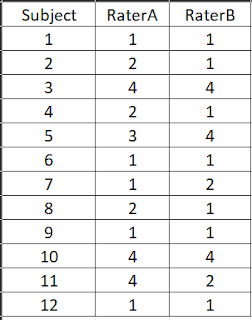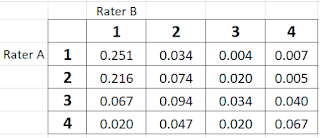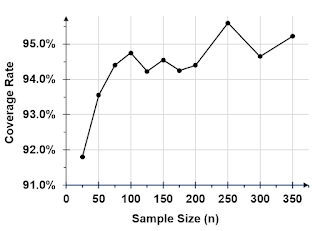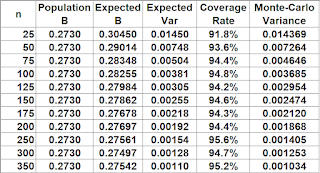## Wednesday, January 11, 2023

### On Bangdiwala's B Coefficient and Its Variance

Bangdiwala's B, is an agreement coefficient for 2 raters, where agreement and disagreement are measured by the areas of some rectangles, and was suggested by Bangwidala S (1985). See Bangdiwala, S.I., Shankar, V. (2013) and Shankar, V., Bangdiwala, S.I. (2014) for more information about this coefficient.

Consider Table 1, an abstract table showing the distribution of $$n$$ subjects by rater and category. Both raters Rater A and Rater B have classified each of the $$n$$ subjects into one of $$q$$ possible categories.Table 1: Distribution of n subjects by rater and by category
Bangdiwala's B statistic, denoted by $$\widehat{B}$$ is given by the following equation:
$\widehat{B}=\frac{\displaystyle\sum_{k=1}^qp_{kk}^2}{\displaystyle\sum_{k=1}^qp_{k+}p_{+k}}, \hspace{3cm}(1)$
where $$p_{kk}=n_{kk}/n$$, $$p_{k+}=n_{k+}/n$$ and $$p_{+k}=n_{+k}/n$$. This coefficient will be rewritten as $$\widehat{B}=\widehat{B}_1/\widehat{B}_2$$, $$\widehat{B}_1$$ and $$\widehat{B}_2$$ being respectively the numerator and the denominator of equation (1).

The variance estimator of Bangdiwala's B that I propose,  is given by the following equation:
$var\bigl(\widehat{B}\bigr) = \frac{2}{\widehat{B}_2^2}\Biggl[2\sum_{k=1}^qp_{kk}^2\bigl(p_{kk}-2\widehat{B}\pi_k\bigr) + \widehat{B}^2\biggl(\sum_{k=1}^q\pi_kp_{k+}p_{+k} + \sum_{k=1}^q\sum_{l=1}^qp_{kl}p_{+k}p_{l+}\biggr)\Biggr]$
where $$\pi_k=(p_{+k}+p_{k+})/2$$, and $$p_{kl}=n_{kl}/n$$.

How do I know this variance estimator works?

Almost all statistics that can be represented as continuous functions of some variables has a probability distribution, which is asymptotically Normal.  That is, as the sample size (in our case the number of subjects) increases, the law of probability associated with the statistic in question looks more and more like the Normal distribution.

Traditionally, the way you demonstrate that a variance formula works, is to start with an initial population of subjects for which the $$B$$ coefficient is known and represents the target parameter to be estimated from smaller samples.  Then, you would conduct a Monte-Carlo experiment by simulating a large number of samples of subjects of various sizes selected from the population. For each simulated sample of subjects, you would compute a 95% confidence interval. This process will produce a long series of 95% confidence intervals. If the variance formula works, approximately 95% of confidence intervals will include the target parameter B and the coverage rate should improve as the sample size increases.

The Monte-Carlo Simulation

I first created the subject population, a file containing 10,000 records the extract of which is shown in Table 2. This file contains ratings from Rater A and Rater B who classified each of the 10,000  subjects into one of 4 categories labeled as 1, 2, 3 and 4. You can download the entire subject population file as an Excel spreadsheet. Bangdiwala's coefficient calculated for this population is $$B=0.2730$$ and is the estimand, or the target population parameter that each sample will approximate.

Note that the classification of subjects to categories was done randomly according the classification probabilities shown in Table  3. For example, both raters would classify a subject into category 1 with a probability $$p_{11}=0.251$$, whereas RaterA and RaterB would classify a subject into categories 1 and 2 respectively with probability $$p_{12}=0.034$$ and so on. An appendix at the end of this post shows an R script used for creating the subject population.

The next step was to select 4,000 samples of a given size from the subject population and use each of them to compute Bangdiwala's B estimate with equation (1), along with its variance estimate. For each sample $$s$$,  I calculated $$\widehat{B}_s$$, its variance $$v_s$$, the associated 95% confidence interval given by $$\bigl(\widehat{B}_s - 1.96\sqrt{v_s};\widehat{B}_s-1.96\sqrt{v_s}\bigr)$$, and a 0-1 dichotomous variable $$I_s$$ that indicates whether the population value $$B=0.273$$ is included into the 95% confidence interval or not. Consequently, for each sample size ($$n$$), a file with 4,000 rows and 4 columns is created. I repeated this process with sample sizes $$n=25, 50, 75, 100, 125, 150, 175, 200, 250, 300, 350$$.

I expect the average of all 4,000 $$I_s$$ values to be reasonably close to 95%, and this coverage rate should get closer and closer to 95% as the sample size increases. It is indeed the case as shown in Figure 1. Even for a small sample size as 25, the coverage rate is already close to 92%. From a sample size of 75 all coverage rates fluctuate between 94% and 95.5%.

The Monte-Carlo simulation program was written in R and can be downloaded. It also contains commands for creating the subject population, although it may not generate the exact same population as the one I used here, because the random number generator will use a different seed.Table 2: Extract of the population of 10,000 subjectsTable 3: Classification probabilities used for creating the subject populationFigure 1: Coverage rates of 95% confidence intervals by sample sizeTable 4: Summary statistics from the Monte-Carlo experiment

References
1. Bangwidala S (1985) A graphical test for observer agreement. Proc 45th Int Stats Institute Meeting, Amsterdam, 1, 307–308
2. Bangdiwala, S.I., Shankar, V. The agreement chart. BMC Med Res Methodol 13, 97 (2013). https://doi.org/10.1186/1471-2288-13-97.
3. Shankar, V., Bangdiwala, S.I. Observer agreement paradoxes in 2x2 tables: comparison of agreement measures. BMC Med Res Methodol 14, 100 (2014). https://doi.org/10.1186/1471-2288-14-100

Appendix: R script for creating the population of 10,000 subjects

pop.size <- 10000
sframe <- sample(x=c(11,12,13,14,
21,22,23,24,
31,32,33,34,
41,42,43,44),
size=pop.size,
prob = c(0.251,0.034,0.004,0.007,
0.216,0.074,0.020,0.005,
0.067,0.094,0.034,0.040,
0.020,0.047,0.020,0.067),
replace = T)

sframe1 <- as.matrix(sframe)
no.neuro <- trunc(sframe1/10)
w.neuro <- sframe1-10*trunc(sframe1/10)
sframe2 <- cbind(sframe1,no.neuro,w.neuro)
sfra <- as_tibble(sframe2) #- This is the subject population -